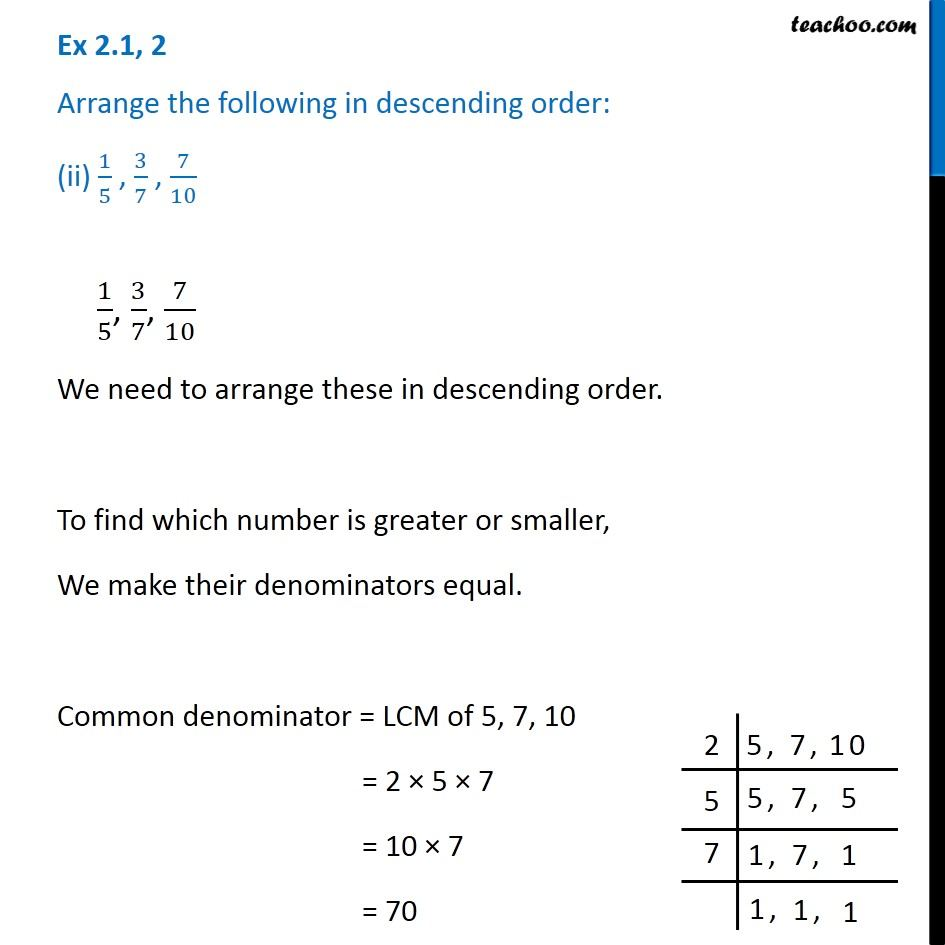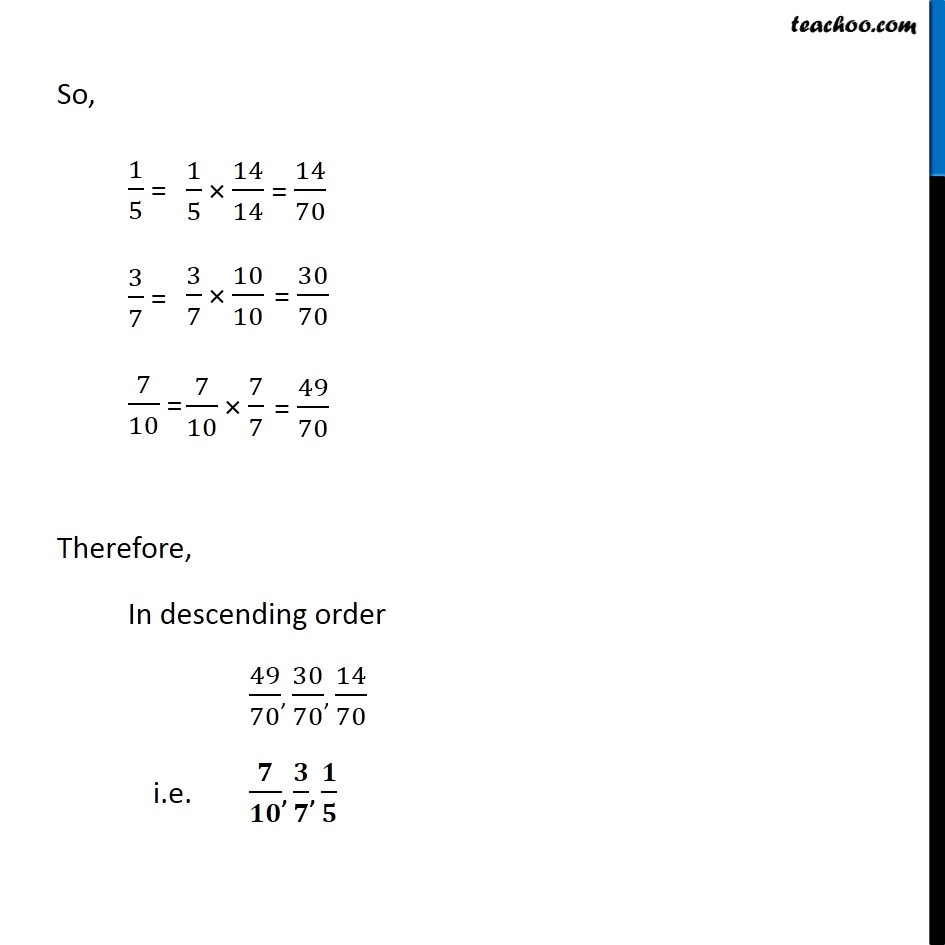Chapter 2 Class 7 Fractions and Decimals
Serial order wiseLearn in your speed, with individual attention - Teachoo Maths 1-on-1 Class

### Transcript

Question 2 Arrange the following in descending order: (ii) 1/5 , 3/7 , 7/10 1/5, 3/7, 7/10 We need to arrange these in descending order. To find which number is greater or smaller, We make their denominators equal. Common denominator = LCM of 5, 7, 10 = 2 5 7 = 10 7 = 70 So, 1/5 = 3/7 = 7/10 = Therefore, In descending order 49/70, 30/70, 14/70 i.e. / , / , /